Satellite Motion - Complete Toolkit

Objectives

1. State and explain the meaning of Kepler’s three laws of planetary motion.
2. Explain the reason that a satellite can be thought of as a projectile that falls around the Earth instead of into it; and to compare and contrast a circular orbit with an elliptical orbit in terms of the force, acceleration and velocity vectors.
3. Use equations to calculate the orbital speed, orbital acceleration and orbital period for a satellite that orbits a central body of known mass a known distance away.
4. Discuss the meaning and the cause of weightlessness and to explain why an orbiting astronaut would experience weightless sensations.
5. Use an energy analysis to explain both the changes in speed and the constant speed of a satellite in an elliptical and a circular orbit.

Interactive Simulations

1.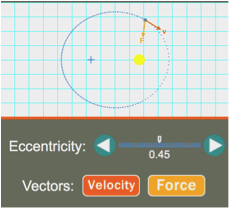Orbital Motion

The Orbital Motion Interactive simulates the elliptical motion of a satellite around a central body. The eccentricity of the orbit can be altered. Velocity and force vectors are shown as the satellite orbits. The Physics Classroom has prepared a classroom-ready activity for use by teachers with their classes.

2.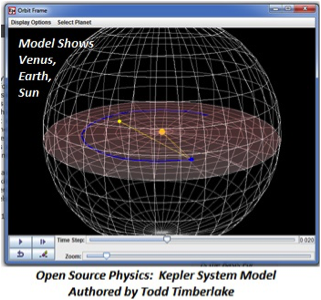Open Source Physics:  Kepler System Model

This robust model will let your students visualize all three of Kepler's Laws. The First and Second Law are especially well-modeled for the beginner, mostly because of the array of tools for viewing. You can show the planet's orbital plane, view the elliptical plane, trace orbits of both Earth and one other chosen planet, show a line-of-sight vector. To explore the Third Law, you can set your own parameters for a user-defined planet. All the computational data was taken from the National Space Science Data Center's fact sheets. The model provides two windows -- one simulates the Sun with Earth and one other planet orbiting; the second shows the view of Sun and Planet against the background stars as see from Earth.

3.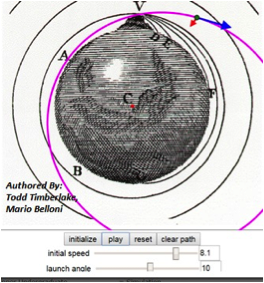Open Source Physics:  Newton’s Mountain Model

As Newton pondered, what would happen if you launched a projectile from a VERY tall mountain on Earth? This HTML sim lets you explore the idea of Newton's Mountain. The model is based on the diagram taken from Newton's "A Treatise on the System of the World", found in the Principia. Newton concluded that a projectile launched horizontally with sufficient speed would orbit Earth, rather than crashing back to the surface. You can set initial speed and launch angle, allow the projectile to pass through Earth, or designate Earth as a point mass.

4.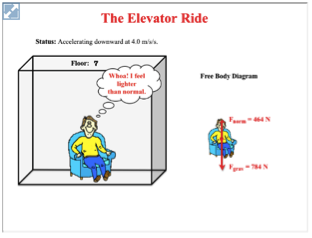The Physics Interactives: Elevator Ride

The Elevator Ride Interactive is a simulation depicting the forces acting upon an elevator rider while ascending and descending. The emphasis on the Interactive is on communicating the sensations of weightlessness and weightiness experienced by a rider. The Physics Classroom has prepared a classroom-ready activity for use by teachers with their students.

Background Information on Space Flight

1. NASA Jet Propulsion Lab:  The Basics of Space Flight

For teachers wanting a deep dive into the fundamentals of space exploration, this is your resource. Packaged by NASA’s Jet Propulsion Lab, this guide for non-physicists covers reference frames, the physics of gravity and planetary orbits, technologies, instrumentation, navigation, spacecraft design, and flight operations from takeoff to encounter.

Videos

1.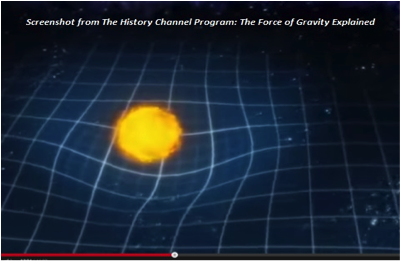The History Channel – The Universe: Gravity

There's lots of video out there dealing with gravity, with lots of grievously wrong information flying around! This well-produced 45-minute video from The History Channel goes at the top of our list for these reasons: 1) It's not juvenile, but comprehensible for high school, 2) Noted scientists present the information in an engaging way, 3) Videography is beautiful, and 4) It knits together concepts of Newtonian gravitation, spacetime curvature, g forces, microgravity, and relationship of gravity and energy. Teachers: Don't let the first 5 minutes fool you - the video quickly escalates to a level appropriate for physics students.

2.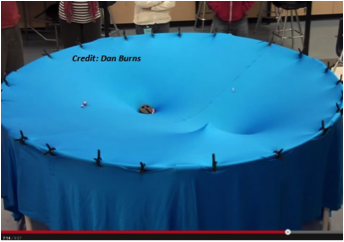Gravity Visualized

How can you build a cheap model to help students visualize gravitation on the cosmic scale? Easy.....spandex, tent poles, binder clips, marbles, ball bearings, and wooden balls. You may have already seen high school teacher Dan Burns' viral video "Gravity Visualized", where he shows how to use the freestanding model. Did you know you can also use it to model the Roche Limit and the tides?

Lab Materials List - Scroll to middle of page!

3.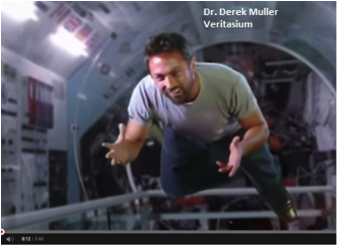Veritasium: Why Are Astronauts Weightless?

Physicist Derek Muller again packs a lot of punch with this short video that debunks the myth that astronauts in the ISS are in a zero-gravity environment. As he explains, the space station orbits Earth at 400 km above the surface, so it is subject to the Law of Universal Gravitation (in other words, it is pulled toward Earth by gravity). The sensation of weightlessness is actually free fall!  So why doesn’t the ISS crash into Earth? It’s traveling at an orbital velocity of 28,000 km/hr, so as it falls, the Earth curves away from it.

Animations

1.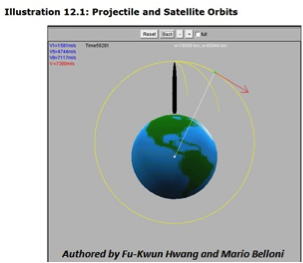Physlet Physics Illustration 12.1: Projectile and Satellite Orbits

There's lots of video out there dealing with gravity, with lots of grievously wrong information flying around! This well-produced 45-minute video from The History Channel goes at the top of our list for these reasons: 1) It's not juvenile, but comprehensible for high school, 2) Noted scientists present the information in an engaging way, 3) Videography is beautiful, and 4) It knits together concepts of Newtonian gravitation, spacetime curvature, g forces, microgravity, and relationship of gravity and energy. Teachers: Don't let the first 5 minutes fool you - the video quickly escalates to a level appropriate for physics students.

2.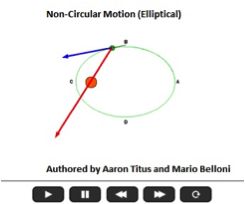Physlet Physics Illustration 12.3: Circular and Noncircular Motion

A green planet orbits an orange star in two animations – one depicting uniform circular motion (circular orbit) and the other showing an elliptical orbit. The velocity vector is shown in blue and the acceleration vector in red. Takeaways: Acceleration in the elliptical orbit is far greater as the planet approaches its perihelion. Second, if there are no other planets or stars nearby, the acceleration of the planet is directed exactly toward the star whether the orbital motion is uniform or not.

3.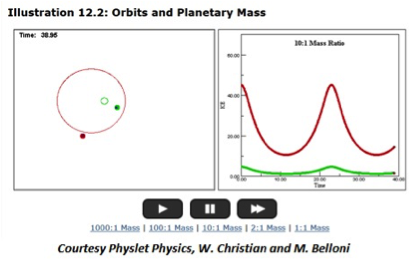Physlet Physics Illustration 12.2:  Orbits and Planetary Mass

This animation closely resembles the Sun/Jupiter system. You can choose a mass ratio of 1000:1, 100:1, 10:1, 2:1, and 1:1. The screenshot shows 10:1.  Kepler’s First Law tells us that the orbit of every planet is an ellipse with the Sun at one of the two foci. But how large does the mass of the Sun have to be to achieve this idealized planetary motion? And what happens to the kinetic energy of the system as a function of time?

4.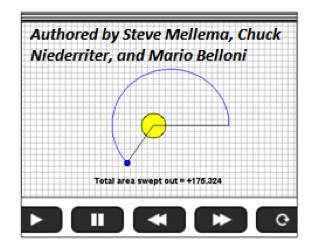Physlet Physics Illustration 12.5:  Kepler’s Second Law

Kepler’s Second Law states that planets sweep out equal areas in their orbits in equal times. This animation displays total area swept out per unit of time. Ask students to choose any time increment (2 years, 3 years, 5 years) and watch the planet sweep out equal areas even though its orbital path is not uniform (it’s elliptical).

5.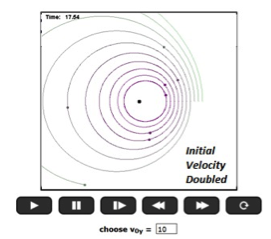Physlet Physics Exploration 12:1:  Different Initial position or Velocity for Planetary Orbits

This exploration with pdf student worksheet shows 10 identical planets orbiting a star. You can change initial position or initial velocity of the planets and watch what happens to the orbits. Takeaways: If the initial velocity is too low, the inner planets will collide with the star. As you increase the initial velocity, the orbits become more uniform (circular). But there’s a tipping point where a too-high initial velocity can send planets shooting off into space, escaping the star’s gravitation.

6.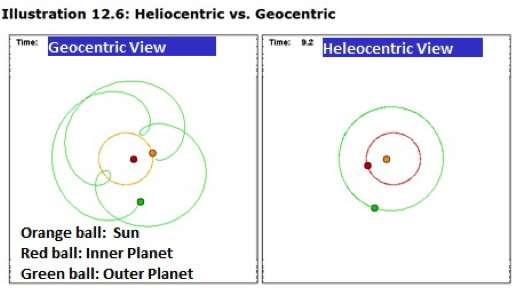Physlet Physics Illustration 12.6:  Heliocentric vs. Geocentric

The motion of the planets as seen from the reference frame of the Sun is pretty simple. But from the perspective of each individual planet (the geocentric reference frame), it gets complicated. This animation explores both reference frames for a system involving a star, an inner planet, and an outer planet. Takeaway: Students will gain appreciation of how difficult it must’ve been for early astronomers to figure out that the planets revolved around the Sun. It also prepares learners to understand that it’s impossible to describe astronomical motion unless you first decide upon a frame of reference.

Labs and Investigations

1. The Physics Classroom, The Laboratory, Satellite Motion Simulation
Students explore circular and elliptical motion of satellites and compare and contrast the direction and magnitude of the force and velocity vector for these two types of orbits.

2. The Physics Classroom, The Laboratory, The Law of Harmonies Analysis
Students are provided data for the orbital radius (in a.u. units) and orbital period (in Earth-year units) for the planets and analyze the data to provide evidence for Kepler's third law of planetary motion.

3. The Physics Classroom, The Laboratory, Jupiter's Moons Analysis
Students analyze orbital period and orbital radius data for several moons of Jupiter in order to determine if Kepler's third law of planetary motion also apply to satellites in general.

4. The Physics Classroom, The Laboratory, Mass of Saturn Analysis
Students use Kepler's third law and orbital data for the moons of Saturn in order to determine the mass of Saturn.

5. The Physics Classroom, The Laboratory, The Mini Drop Lab
Students use a force scale and a 1-kg mass to explore the strength of the upward force on an object as it free falls and as it is brought to a stop after free fall.

Video Analysis Exercise

1.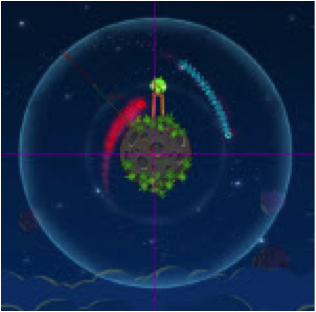Tracker Video Analysis:  Angry Birds in Space

What type of force is exerted on Angry Birds in Space? This resource set explores the physics behind the video game designed by Rovio, makers of Angry Birds. The package includes zip files of Angry Birds in Space video clips and Tracker files that allow you to perform precise video analysis of the motion. Also included is the blog entry that goes with this topic, a video, and a link to download and run the free Tracker analysis tool. Even if you can't access Java in computer lab, author Rhett Allain's pdf document gives you all the background you need to set it up yourself in the classroom.

Elsewhere on the Web

1.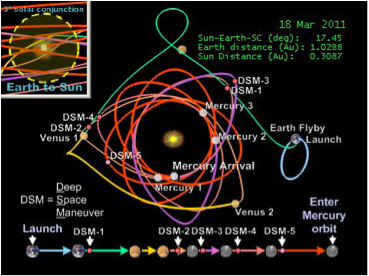Classroom Lesson Module:  Give Me A Boost – Gravity Assist

What type of force is exerted on Angry Birds in Space? This resource set explores the physics behind the video game designed by Rovio, makers of Angry Birds. The package includes zip files of Angry Birds in Space video clips and Tracker files that allow you to perform precise video analysis of the motion. Also included is the blog entry that goes with this topic, a video, and a link to download and run the free Tracker analysis tool. Even if you can't access Java in computer lab, author Rhett Allain's pdf document gives you all the background you need to set it up yourself in the classroom.

Minds On Physics Internet Modules:

The Minds On Physics Internet Modules are a collection of interactive questioning modules that target a student’s conceptual understanding. Each question is accompanied by detailed help that addresses the various components of the question.
1. Circular Motion and Gravitation, Ass’t CG8 -  Satellite Motion
2. Circular Motion and Gravitation, Ass’t CG9 -  Weightlessness
3. Circular Motion and Gravitation, Ass’t CG10 -  Kepler's Laws of Planetary Motion

Concept Building Exercises:

1. The Curriculum Corner, Circular Motion and Gravitation, Satellite Motion
2. The Curriculum Corner, Circular Motion and Gravitation, Weightlessness
3. The Curriculum Corner, Circular Motion and Gravitation, Kepler's Laws of Planetary Motion

Problem-Solving Exercises:

1. The Calculator Pad, ChapterGoesHere, Problems #19 - #27

Science Reasoning Activities:

1. Kepler's Law of Harmonies

Common Misconception:

1. Satellites as Projectiles

Students at first find it difficult to believe that artificial satellites are projectiles. They figure that there must be a force other than gravity acting upon them. While such satellites may on occasion use thrust to fine-tune their orbits, they are essentially projectiles that fall towards the Earth relative to their inertial path without ever getting any closer to the earth. When provided the logic behind this concept, they tend to buy in to the essential nature of satellite motion.

2. Causes of Weightlessness

Ask a student why astronauts feel weightless and you will undoubtedly hear the response that there is no gravity acting upon them. A good deal of poor word choice for numerous years has likely contributed to this misconception. References to "microgravity" and "zero-gravity" may not be the best choice of terms to describe the gravitational conditions of orbiting astronauts as students assign different meaning to these terms as was intended by those who use them. The result is often some very strong conceptions about what is meant by weightlessness. It is important to explain to students that weightlessness does not result when there is no gravity force but rather results when the only force present is the gravity force.

Standards:

A. Next Generation Science Standards (NGSS)

Performance Expectations
• Forces and Motion HS-PS2-4:  Use mathematical representations of Newton’s Law of Gravitation to describe and predict the gravitational forces between objects.
• Forces and Motion  HS-PS2-2:  Use mathematical representations to support the claim that the total momentum of a system of objects is conserved when there is no net force on the system.
• Energy  HS-PS3-1:  Create a computational model to calculate the change in the energy of one component in a system when the change in energy of the other component(s) and energy flows in and out of the system are known.

Disciplinary Core Ideas
• Forces and Motion-Types of Interactions    HS-PS2.B.i     Newton’s Law of Universal Gravitation and Coulomb’s Law provide the mathematical models to describe and predict the effects of gravitational and electrostatic forces between objects.
• Forces and Motion-Types of Interactions     HS-PS2.B.ii   Forces at a distance are explained by fields (gravitational, electric, and magnetic) permeating space that can transfer energy through space. Magnets or electric currents cause magnetic fields; electric charges or changing magnetic fields cause electric fields.
• Forces and Motion    HS-PS2.A.ii    Momentum is defined for a particular frame of reference; it is the mass times the velocity of the object.
• Forces and Motion    HS-PS2.A.iii    If a system interacts with objects outside itself, the total momentum of the system can change; however, any such change is balanced by the changes in the momentum of objects outside the system.
• Conservation of Energy    HS-PS3.B.i    Conservation of energy means that the total change of energy in any system is always equal to the total energy transferred into or out of the system.
• Conservation of Energy    HS-PS3.B.ii    Mathematical expressions, which quantify how the stored energy in a system depends on its configuration and how kinetic energy depends on mass and speed, allow the concept of conservation of energy to be used to predict and describe system behavior.
• Relationship Between Energy and Forces    HS-PS3.C.i   When two objects interacting through a field change relative position, the energy stored in the field is changed.

NGSS Engineering and Technology Standards  (ETS)
• High School-ETS1.A.i      When evaluating solutions it is important to take into account a range of constraints including cost, safety, reliability, and aesthetics and to consider social, cultural, and environmental impacts.
• High School-ETS1.A.ii   Both physical models and computers can be used in various ways to aid in the engineering design process. Computers are useful for a variety of purposes, such as running simulations to test different ways of solving a problem or to see which one is most efficient or economical; and in making a persuasive presentation to a client about how a given design will meet his or her needs.

Crosscutting Concepts
Scale, Proportion, and Quantity
• High School:  The significance of a phenomenon is dependent on the scale, proportion, and quantity at which it occurs.

Systems and System Models
• High School: When investigating or describing a system, the boundaries and initial conditions of the system need to be defined and their inputs and outputs analyzed and described using models.
• High School:  Models can be used to predict the behavior of a system, but these predictions have limited precision and reliability due to the assumptions and approximations inherent in models.

Nature of Science:  Order & Consistency in Natural Systems
• High School:  Scientific knowledge is based on the assumption that natural laws operate today as they did in the past and they will continue to do so in the future.
• High School:  Science assumes the universe is a vast single system in which basic laws are consistent.
• High School:  Science assumes that objects and events in natural systems occur in consistent patterns that are understandable through measurement and observation.

Science and Engineering Practices
Practice #1: Analyzing and Interpreting Data
• High School:  Analyze data using tools, technologies, and/or models (e.g., computational, mathematical) in order to make valid and reliable scientific claims

Practice #2: Developing and Using Models
• High School:  Develop and use a model based on evidence to illustrate the relationships between systems or between components of a system.  (Strong alignment)
• High School:  Use a model to provide mechanistic accounts of phenomena.  (Strong alignment)

Practice #3: Planning and Carrying Out Investigations
• High School:  Plan and conduct an investigation individually and collaboratively to produce data to serve as the basis for evidence.

Practice #4: Analyzing and Interpreting Data
• High School:  Construct an explanation based on valid and reliable evidence obtained from a variety of sources (including students’ own investigations, models, theories, simulations) and the assumption that theories and laws that describe the natural world operate today as they did in the past and will continue to do so in the future.

Practice #5: Using Mathematics and Computational Thinking
• High School:  Create or revise a computational model or simulation of a phenomenon, process, or system. (Strong alignment)
• High School:  Use mathematical representations of phenomena or design solutions to describe and/or support claims and/or explanations. (Strong alignment)

B. Common Core Standards for Mathematics – Grades 9-12

Standards for Mathematical Practice:
• Reason abstractly and quantitatively
• Model with mathematics
• Make sense of problems and persevere in solving them

Quantities
• High School N-Q.1: Use units as a way to understand problems and to guide the solution of multi-step problems; choose and interpret units consistently in formulas; choose and interpret the scale and the origin in graphs and data displays.

Algebra
• High School A-SSE.1.     Interpret parts of an expression, such as terms, factors, and coefficients.
• High School A-SSE.2      Use the structure of an expression to identify ways to rewrite it.
• High School A-CED.2      Create equations in two or more variables to represent relationships between quantities
• High School A-CED.4      Rearrange formulas to highlight a quantity of interest, using the same reasoning as in solving equations.

Functions
• High School F-IF.4     For a function that models a relationship between two quantities, interpret key features of graphs and tables in terms of the quantities, and sketch graphs showing key features given a verbal description of the relationship.
• High School F-IF.6     Calculate and interpret the average rate of change of a function (presented symbolically or as a table) over a specified interval. Estimate the rate of change from a graph.

• High School F-LE.1.b     Recognize situations in which one quantity changes at a constant rate per unit interval relative to another.
• High School F-LE.5     Interpret the parameters in a linear or exponential function in terms of a context.

Geometry
• High School G-C.5     Derive using similarity the fact that the length of the arc intercepted by an angle is proportional to the radius, and define the radian measure of the angle as the constant of proportionality.
• High School G-GPE.3    Derive the equations of ellipses and hyperbolas given the foci, using the fact that the sum or difference of distances from the foci is constant.

C. Common Core Standards for English/Language Arts (ELA) – Grades 9-12
Key Ideas and Details
• High School RST11-12.3      Follow precisely a complex multistep procedure when taking measurements or performing technical tasks; analyze the specific results based on explanations in the text.
• High School RST.11-12.2     Determine the central ideas or conclusions of a text; summarize complex concepts, processes, or information presented in a text by paraphrasing them in simpler but still accurate terms.

Integration of Knowledge and Ideas
• High School RST.11-12.7      Integrate and evaluate multiple sources of information presented in diverse formats and media (e.g., quantitative data, video, multimedia) in order to address a question or solve a problem.
• High School RST.11-12.9      Synthesize information from a range of sources (e.g. texts, experiments, simulations) into a coherent understanding of a process, phenomenon, or concept.

Range of Reading and Level of Text Complexity
• High School RST.11-12.10      By the end of Grade 10, read and comprehend science/technical texts in the Grade 9-10 text complexity band independently and proficiently.